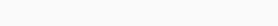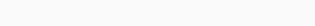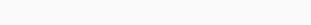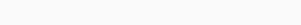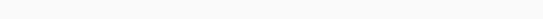# Pre Algebra Fractions

With Cymath Pre Algebra Fractions, knowing fractions is the first step toward becoming an expert in mathematics. Throughout this guide, we’ll explore Pre-Algebra: Fractions, explore their importance, and provide practice problems to help you master it. I will lead you through the logical aspects of fractions as your math expert.

## The Significance of Fractions in Pre-Algebra:

As a student of Pre-Algebra, you’ll frequently encounter fractions. Here’s why they’re so important:

### Real-world Application:

You use fractions in everyday life, from splitting pizza between friends to calculating discounts during shopping. Mastering fractions prepares you for life.

### Foundation for Algebra:

It is essential for future math courses to understand fractions in order to solve algebraic equations, expressions, and inequalities.

### Mathematical Operations:

It is easy to add, subtract, multiply, and divide numbers when you are proficient in fractions.

### Problem Solving:

Many word problems involve fractions. Being adept at fractions equips you to solve a wide range of math problems effectively.

## Logical Aspects of Fractions:

### To excel in Pre-Algebra:

Fractions, you need to grasp some logical concepts:

### Numerator and Denominator:

A fraction consists of a numerator (top number) and a denominator (bottom number). The numerator represents the part you have, and the denominator represents the whole.

### Equivalent Fractions:

Fractions that represent the same value but are written differently are called equivalent fractions. They have the same ratio of numerator to denominator.

Adding and subtracting fractions require a common denominator, which can be found by finding a multiple of the denominator.

### Multiplying Fractions:

For multiplying fractions, multiply the numerators together and the denominators together to get the new numerator.

### Dividing Fractions: